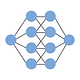# Intuitive Deep Learning Part 4: Deep Reinforcement Learning

## How do we play Atari games just from pixel values? A gentle introduction to Deep Reinforcement Learning!

If you’ve read the news on Artificial Intelligence, you might have come across some of its impressive feats in playing games (from Atari games to DotA and Starcraft). These AI systems are often powered by reinforcement learning, and in this post, we’ll see how we can apply neural networks to reinforcement learning.

Series Note: This is the fifth and last post of the introductory series. I hope you’ve enjoyed this series — please and follow this publication to get more of this kind of content!

: In part 1, we had an introduction to neural networks and how to make them work. We started off in Part 1a with a high-level summary of what Machine Learning aims to do:

Machine Learning specifies a template and finds the best parameters for that template instead of hard-coding the parameters ourselves. A neural network is simply a complicated ‘template’ we specify which turns out to have the flexibility to model many complicated relationships between input and output. Specifying a loss function and performing gradient descent helps find the parameters that give the best predictions for our training set.

Our neural networks have thus far been applied to many applications — ranging from image detection to machine translation, which we’ve covered in Part 2 and Part 3 of the introductory series. In this post, we will see how AI systems can play games through reinforcement learning.

Thus far in our series, we have looked at training examples consisting of an input and a correct output our model is supposed to predict. Dealing with this type of training data is called supervised learning. In applications such as playing games, however, we don’t see the same pattern of an input and a correct output our model is supposed to predict.

Nevertheless, you might have seen on the news how Deep Learning is still behind many of the stunning achievements of AI systems. This includes:

• DeepMind, an AI company, built an AI system that was able to play a series of Atari games using just the pixel values. This means that all the AI was able to see were the very pixels we humans can see, and no knowledge of the game rules and structure was given to the AI beforehand. The same AI played a whole host of Atari games, and beat human performance in many of them. Here’s an example of Google DeepMind playing Atari Breakout by using reinforcement learning:
• DeepMind also created AlphaZero, an AI system which has convincingly beaten the top humans (and other AI systems) in complicated games such as Chess, Shogi and Go. Go is a very complicated game, with the total possible board configurations being on the order of 10¹⁷⁴. Merely a few years ago, there were many skeptics that an AI would beat the human world champion in Go. Yet now we have AI systems that have done so, and these systems are powered by reinforcement learning.

AlphaZero could start in the morning, playing completely randomly, and then by tea, be a super-human level and by dinner, it’d be the strongest chess entity that it has ever been. — Demis Hassabis, CEO of DeepMind

So what is Reinforcement Learning? Reinforcement Learning is about learning from experience by interacting with the world rather than learning from example.

In Reinforcement Learning, we call our AI system the agent. The agent makes observations about the environment (state), then interacts with the environment by taking actions. The environment gives feedback to the agent in the form of rewards, which we wish to maximize.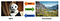Agent interacts with the environment by taking actions after seeing what the state is. The environment gives feedback to the agent in the form of rewards.

In a game of Chess, for example, the AI system (agent) observes the chess board (state) and then interacts with the chess board (environment) by making valid chess moves (actions). The other player could make a move as well, changing the environment. The reward that is given to the agent occurs when the agent has won the game (a negative reward could be given when the agent has lost the game). The aim of the AI agent should be to maximize the reward it receives by winning the game.

Note that this is a different paradigm from what we have been used to in the other parts of the introductory series. Previously, in Supervised Learning, we had access to an existing dataset and we can learn the complicated function mappings, for example between X-ray images and its diagnoses. In the Reinforcement Learning paradigm, we are continuously interacting with the environment and the feedback or reward on our actions might not be immediate. A genius / disastrous chess move might manifest a win or loss in our chess game only after many many moves.

This interaction with the environment in Reinforcement Learning presents unique dynamics we have not seen before. For example, we might want to explore the environment to see how the world works rather than always exploit what we currently think is the best strategy. If we want to watch a movie, we may explore watching a new movie we’ve not seen or we may exploit our current knowledge and watch our favorite movie again.

: Reinforcement Learning is about learning from experience by interacting with the environment through observing the state and taking actions to maximize a numerical reward.

The first question we must confront in this paradigm is: What exactly are we trying to learn? After all the learning has been done by our RL agent, what is the output? In supervised learning, we wanted an accurate mapping between input and output; but what’s the “end goal” for all the learning done in reinforcement learning?

At the end of all our learning, we want the RL agent to specify what action it would like to take when it observes a state. We call this a policy: a mapping between states and the actions our agent will take. In chess, the RL agent should tell me what the next chess move should be when it sees that particular chess board. In robotics, the robot should know how to move (left, right, forward, backward) when it observes the environment around it.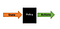We want the RL agent to learn a policy that specifies an action the agent should take based on the state the agent observes

A good policy is one that achieves a high reward from its environment when executed. The chess agent which makes intelligent moves for any chess position it finds itself in will likely gain the reward from winning the game at the end. A bad policy, conversely, is one that achieves a low reward from its environment when executed. Our RL algorithm thus seeks to learn the best policy based on some defined reward function.

The reward and the reward function is something that you have to define at the offset. In chess, we might specify the reward to only be based on win/lose at the end of the game; or we might specify a small negative reward (penalty) for each move, thereby encouraging the agent to end the game quicker rather than slower. A reward function specifies how to combine the rewards at every time step. A simple reward function would be to simply sum up all the rewards at each time step. Alternatively, we can say that rewards further in the future matter less to us than immediate rewards. We call this discounting. These are value judgments you have to make based on what you want the AI agent to perform towards.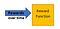Reward function combines the rewards over time to get an overall reward that we want to maximize

To sum it all up in one figure, this is what our RL agent is trying to do: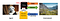In Reinforcement Learning, the agent learns a policy to maximize the reward function. The policy takes the state it observes and specifies an action it ought to do. This action affects the environment in some way which gives the agent rewards, and the rewards over time are combined through the reward function.

: In RL, we want to learn the best policy (mapping between states to actions) for our agent which maximizes some reward function.

The question remains: how should we learn the best policy that maximizes our reward function?

To determine the appropriate reinforcement learning algorithm to use, we have to understand more about the problem we are trying to solve. For example,

• Do we know exactly how the world works? That is, do we know exactly how the state will transition to other states and how rewards are given per each state? If we have some kind of model of how the world works, our AI agent can use that to plan ahead; if not, we will have to learn via trial-and-error.
• How many possible states are there? If there are only a few states, we might be able to create an explicit list of actions for every state. If there are too many states to do so, we have to apply more approximate methods.

A fuller and more comprehensive treatment of the different RL algorithms will be the topic of a future post. Nevertheless, for this post, we will zoom in on the problem of learning how to play Atari games from pixel values to see how neural networks can apply to Reinforcement Learning problems. Let’s answer the questions above for this task:

• We do not know how the world works. Or at the very least, we cannot use that knowledge in this task. The aim of playing different Atari games from pixel values is to learn how to play the games even without any prior knowledge of how the game works.
• Since we take the pixel values as the state, the question is: how many possible states can we observe? Suppose the screenshot is 80 pixels * 80 pixels = 6400 pixels. (In reality, we downsize the actual screen to 84 pixels * 84 pixels.) Even if each pixel can only take two states, black and white, the total number of states is 2⁶⁴⁰⁰. Furthermore, we often take four frames in succession so that we get an idea of motion, so the total number of states is really 2²⁵⁶⁰⁰. This is huge!

Keep these restrictions in mind as we explore Deep Q-Learning, the algorithm that we use here. But before we get into the Deep Q-learning, let’s explore the foundations of Q-learning first.

To figure out a policy, we typically have to first figure out how valuable it is to be in each state. We call this a value function. In chess, we can imagine the chess board where you have checkmated the opponent to be a very valuable state, since it gives you the reward for winning. A prior position that will lead to that checkmate will be very valuable, even if you have not gotten a reward for that position yet. A value function will tell you the value for each state, in anticipation of future rewards down the road as well.

Suppose we have already somehow calculated our value function. The optimal policy we take will be pretty obvious: look through all your actions and see what states your actions will lead you to. Then, simply pick the action that will lead you to the state of highest value! In chess, it would be to pick the action that will lead to a more advantageous chessboard position, as defined by the value of that state. This is how we might approach some reinforcement learning problems.

Let’s go through a simple example, where we are a robot who can move left or right (actions) in a map that is divided into five positions (states):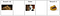Robot and the environment he is in. Two steps to the left of the robot there is a pile of gold coins which will give him a reward of 10, and two steps to the right of the robot there is a pile of chocolate coins which will give him a reward of 1.

When the robot reaches either reward, the game stops. Suppose we somehow calculated a value function for each state that looks like this:

The value function tells the robot how valuable being in each position is. Now that he is in the middle, should he move left or move right? Since the square left to him gives him a value of 9 and the square right to him gives him a value of 4, he should move left!

If we do this for all states, we can get the policy (orange arrows) from the value function: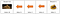A policy which specifies the action to take based on the value of the next state if the action was taken

The policy specifies an action to take no matter where the robot is.

However, remember that our first restriction is that we do not know how the world works. In other words, we do not know how our actions will lead to the next state. Even if we had a value for each and every state, it does not tell us anything about what actions we should take. So this value function for each state isn’t as helpful in our situation. Instead, we need to have a value for the state and each action you can take in that state. This value for each state and action is called the q function.

In our robot example, suppose that the robot malfunctions and each action might teleport him randomly to each square. How the robot is teleported you have no idea, since you don’t know how the world works. In this case, we need to say what the value of taking each action is in each state, rather than the value of being in a state. Suppose we manage to find it, and it looks something like this:

Note that once we reach any of the reward states, the game is over so there is no left or right actions at the reward squares. The policy (green arrows) we choose is simply the action with the highest value in the state where we can make an action.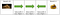The policy is the action in that state which gives the highest value

The above specification is called the Q-table, where Q represents the Quality of action in each state. The Q-table tells us the q value for each state and each action because we do not know how our actions will lead to the next state.

When we do not know how the world works, we can specify a q function, which is a value for each state and action. Our policy then takes the most valuable action in whatever state the agent is in.

Let’s go back to the problem of playing Atari games. Having a Q-table has addressed the first restriction, that is, we do not know how the world works. With a Q-table, we don’t need to know how the world works because we specify a q value for every action in each state. But suppose we want to list a Q-table for playing Atari games. Recall from above that there are 2²⁵⁶⁰⁰ possible states in our problem. Even if there are only four possible actions, listing explicitly a value for every possible state-action pair is simply infeasible in terms of memory storage as well as the time and data needed to fill them accurately! How then do we go about tackling this problem?

What is a Q-table really? It’s an explicit list of actions for every state. In some ways, you can think of it as a little machine. For our simplistic example, you give this machine the state that you are in, and it looks up this Q-table and tells you a value for each action in that state: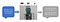You give a machine the state you’re in, he looks up the Q-table and tells you the q value for each action that you take in the state

However, as we mentioned, this breaks down if we want to apply this to play Atari games since we need an enormously huge Q-table: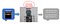If we want to apply the same method for Atari games, we find that we need an enormously huge Q-table, which is not feasible

I wonder, though, if this input-output pattern seems familiar by now. The robot is simply a that takes in something as input (the state you’re in) and spits out an output (a q value for each action in that state). Instead of using a table for this complicated function, why not use neural networks which are in essence complicated functions as well!

Deep Q-Learning looks a bit like this: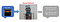You give the neural network a state that you’re in, it processes it through the neural network and outputs for you the q value for each action you take in that state

And here is where Deep Learning comes in! Deep Learning allows us to learn some complicated function which maps the state we see to the q values for each action we can take in that state. Then, our policy is to simply take the action that corresponds to the largest of those q values!

Now, we’ve learnt previously from Part 2 that a special type of neural network called Convolutional Neural Networks (CNNs) are more appropriate to deal with images. Since the input state in our case is indeed image data, we can use CNNs to represent the complicated function to map images to q values!

: When there are too many states, we can use a neural network for the q function to map between the state and the q value for each action we can take.

At this point, one burning question should remain that we have thus far left unanswered: How do we learn this q function? If we have a neural network, how do we train this neural network exactly? What do we use as our training dataset?

Here, we introduce a concept called experience replay. Experience replay simply means we play the game and we record our experiences into a dataset. We then randomize the order of this dataset and use it as our training dataset to train the neural network later on.

Each data point in this dataset contains four elements:

• current state,
• action taken,
• immediate reward observed,
• next state.

Remember from the earlier posts in this introductory series (Part 1a, Part 1b) that we need a ground truth label and a loss function, which tells us how far away our current prediction is from the ground truth. Training this neural network is no different! We need a ground truth label and a loss function in order to use gradient descent to find the optimal values for our parameters in the neural network.

The loss function is the square of how far our value is from the ground truth: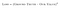The loss function is the square of how far our value is from the ground truth

So for each of our data points in our replay dataset, we input in the current state and the action taken to get an estimate of the q value. We then compare this with the ground truth to get the loss function. All that we are missing in this puzzle now is the ground truth.

To find this ground truth, we consider two possible scenarios (based on the subsequent state, which is a data point that we have collected):

• Scenario 1: The next state is the end of the game.
• Scenario 2: The next state is the end of the game.

In Scenario 1, we’ve reached the end of the game. So we don’t need to consider any future rewards, only the immediate rewards. The ground truth in this case is just the immediate reward we’ve collected at that point.

Let’s use an example of chess. Suppose we have this data point: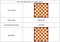One of the data points where we have the current state, the action taken, the immediate reward given and the next state

Since the next state is a checkmate and the game ends, we don’t have to worry about any future moves. The value of the action taken (Rook to A8) should thus be given the value of the immediate reward. In other words, the ground truth for the current state and the action Rook to A8 should have a q value of +100. That’s our ground truth. If that’s not what our neural network predicted at the start, then we have a loss based on how far away our prediction is from the q value, and we use gradient descent to minimize that loss.

Now, let’s consider Scenario 2, where we have yet to reach the end of the game. Then, our ground truth value is calculated like this:Calculation of our ground truth in Scenario 2 where the game has not ended. Gamma refers to the discount factor, which says that values for the future are not as valuable as immediate rewards.

In Scenario 2, we must consider that the next state we reach from that action might not be as valuable (e.g. a more compromising chess position). We must incorporate that in by giving the value of the next state together with the immediate reward. If we value the future less, we include a discount factor, gamma, to account for that temporal valuation.

But how do we know the value of the next state? We use our current estimate by finding the maximum q value of all actions in the next state.

Now, this is merely an estimate, since our q values are not perfect yet (since we haven’t had a fully trained model). But the intuition here is that as our q values get more and more accurate from Scenario 1, this translates to more and more accurate calculations here in Scenario 2.

Imagine that the next state here was the pre-checkmate state in our example above. You could reason that the pre-checkmate state (and the optimal action Rook to A8) will get updated closer and closer to +100 because of Scenario 1. But since the pre-checkmate state is our next state in Scenario 2, the value of the next state we calculate will also get closer and closer to what it’s supposed to be.

In case we’ve missed the bigger picture, let’s recap what we’ve been trying to do: we split into these two scenarios to calculate the ‘ground truth’ value for the state-action pair we’ve seen in our experience replay database. And now that we have our ground truth and our training dataset, we apply all that we’ve learnt in Part 1a and Part 1b: Having this ground truth value and putting it in our loss function helps us use gradient descent to learn our neural network that maps state-action pairs into their q values. And this neural network is exactly what we need when the number of possible states are way too large, as in the case of playing Atari games!

There are a few details that I have glossed over, but I want to emphasize one of them in particular. When we collect our replay dataset by simulating play, what strategy do we use to play? Here, we come back to the idea of exploration vs exploitation that we talked about earlier. We could pick the best action based on what we know so far to try and win the game, but that means that some actions will never get explored! One simple way to encourage exploration is to specify with some probability that a random action will be taken. We call this a ε-greedy approach.

: To train our neural network, we play the game and record our experiences into a replay dataset, where each data point captures the current state, the action taken, the immediate reward observed, and the next state. We then use these data points to train our neural network by first calculating the ground truth value, which feeds into our loss function that tells us how far away our prediction is from the ground truth. We then apply gradient descent to minimize the loss function (Part 1a and Part 1b).

: Reinforcement Learning is about learning from experience by interacting with the environment through observing the state and taking actions to maximize a numerical reward. In RL, we want to learn the best policy (mapping between states to actions) for our agent which maximizes some reward function. When we do not know how the world works, we can specify a q function, which is a value for each state and action. Our policy then takes the most valuable action in whatever state the agent is in. When there are too many states, we can use a neural network for the q function to map between the state and the q value for each action we can take. To train our neural network, we play the game and record our experiences into a replay dataset, where each data point captures the current state, the action taken, the immediate reward observed, and the next state. We then use these data points to train our neural network by first calculating the ground truth value, which feeds into our loss function that tells us how far away our prediction is from the ground truth. We then apply gradient descent to minimize the loss function (Part 1a and Part 1b).

In this post, we saw how neural networks can be used for Reinforcement Learning to play Atari games just from pixel values alone. Reinforcement Learning is a much larger field than just the concepts we’ve covered here today, and I hope to write more on some of the RL concepts that I did not get to cover in this post.

And with that, we’ve reached the end of the introductory series of Intuitive Deep Learning! In this journey, we talked about how neural networks work and some of the nitty-gritty details needed (Part 1a and Part 1b), then applied them to the applications of Computer Vision (Part 2) and Natural Language Processing (Part 3) before talking about how they feature in playing Atari games in Deep Reinforcement Learning.

I hope you have enjoyed the series thus far, please clap and follow and share if you have found it beneficial! There will be more and more posts on this publication on more advanced topics, as well as some beginner-friendly coding companion that you can use to get your hands dirty. So stay peeled for what’s to come!

Written by

Written by

## Joseph Lee Wei En

#### I want to make Deep Learning concepts as intuitive and as easily understandable as possible by everyone! Follow my publication to learn more!